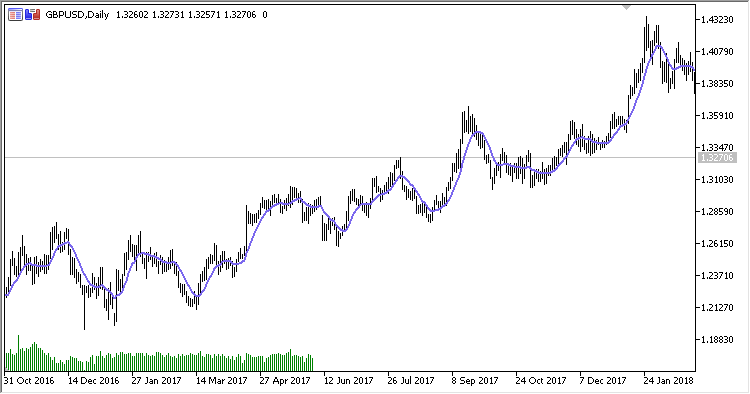Interesting script?
So post a link to it -
let others appraise it

You liked the script? Try it in the MetaTrader 5 terminal# Avg_MA - indicator for MetaTrader 5

Views:
1033
Rating:
Published:
2018.10.25 17:56

Average from MA indicator calculates the average value from the specified number of moving averages, whose periods are calculated as an increment to the base МА period

It has six input parameters:

• First MA period - МА calculation base period
• Method - МА calculation method
• Applied price - МА calculation price
• Count - number of calculated МАs
• Coefficient of increment - increment ratio of calculated MA periods
• Incremental method - increment method
• Multiplication

Example of calculation with values:

First MA period = 5
Coefficient of increment = 2
Count = 5

• If Incremental method = Addition:
AvgMA=(MA(5)+MA(7)+MA(9)+MA(11)+MA(13))/5
• If Incremental method=Multiplication:
AvgMA=(MA(5)+MA(10)+MA(20)+MA(40)+MA(80))/5

where:

MA - MovingAverage(Applied price,Method) with calculated periodTranslated from Russian by MetaQuotes Software Corp.
Original code: https://www.mql5.com/ru/code/22420Generalized DEMA

Generalized DEMAConnors_RSI

Connors RSI indicatorGeneralized double DEMA

Generalized double DEMAMA_Trend

MA Trend indicator